StatLect

# Marginal probability mass function

Consider a discrete random vector, that is, a vector whose entries are discrete random variables. When one of these entries is taken in isolation, its distribution can be characterized in terms of its probability mass function. This is called marginal probability mass function, in order to distinguish it from the joint probability mass function, which is instead used to characterize the joint distribution of all the entries of the random vector considered together.## Definition

The following is a more formal definition.

Definition Letbediscrete random variables forming arandom vector. Then, for each, the probability mass function of the random variable, denoted by, is called marginal probability mass function.

Remember that the probability mass functionis a functionsuch thatwhereis the probability thatwill be equal to.

By contrast, the joint probability mass function of the vectoris a functionsuch thatwhere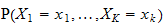is the probability thatwill be equal to, simultaneously for all.

## How to derive it

Denote bythe support of(i.e., the set of all values it can take). The marginal probability mass function ofis obtained from the joint probability mass function as follows: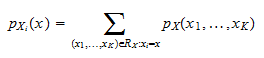where the sum is over the setIn other words, the marginal probability mass function ofat the pointis obtained by summing the joint probability mass functionover all the vectors that belong to the supportand are such that their-th component is equal to.

## Example

Letbe arandom vector with support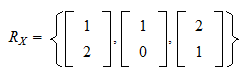and joint probability mass function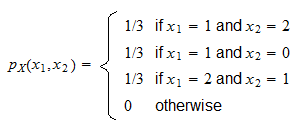The marginal probability mass function ofevaluated at the pointisWhen evaluated at the pointit is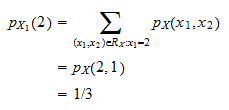For all the other points, it is equal to zero. Therefore, we have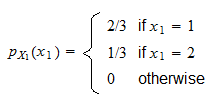## More details

A more detailed discussion of the marginal probability mass function can be found in the lecture entitled Random vectors.